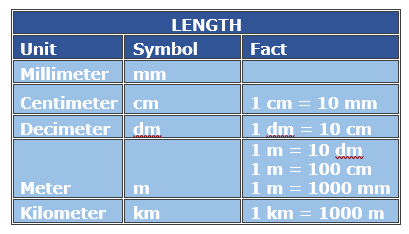# Metric Conversion with Decimal Values: Two-Step Problem

The basic metric units are meters (for length), grams (for mass or weight), and liters (for volume).

The different tables help in converting among these metric units of length, weight and capacity.

In this lesson, we solve problems on conversion among metric units involving two-steps and decimal values.Fill in the blank to make the conversion true.

4500000 millimeters = __ kilometers

### Solution

Step 1:

We know that

1 kilometer (km) = 1000 meters (m)

1 meter (m) = 1000 millimeters (mm)

Step 2:

4500000 millimeters = 4500000 mm × 1 m/1000 mm × 1 km/1000 m

= 4.5 km

So, 4500000 millimeters = 4.5 kilometers

Fill in the blank to make the conversion true.

3.99 kilograms = __ milligrams

### Solution

Step 1:

We know that

1 kilogram (kg) = 1000 grams (g)

1 gram (g) = 1000 milligrams (mg)

Step 2:

3.99 kilograms = 3.99 kg × 1000 g/1 kg × 1000 mg/1g = 3990000 mm

So, 3.99 kilograms = 3990000 milligrams

Fill in the blank to make the conversion true.

3356000 milliliters = 3.356 kiloliters

### Solution

Step 1:

We know that

1 kiloliter (kl) = 1000 liters (l)

1 liter (l) = 1000 milliliters (ml)

Step 2:

3356000 milliliters = 3356000 ml × 1 l/1000 ml × 1 kl/1000 l = 3.356 kl

So, 3356000 milliliters = 3.356 kiloliters